GeeksforGeeks App
Open AppBrowser
Continue

## Related Articles

In statistics, graph plays an important role. With the help of these graphs, we can easily understand the data. So in this article, we will learn how to represent the cumulative frequency distribution graphically.

### Cumulative Frequency

The frequency is the number of times the event occurs in the given situation and the cumulative frequencies are the sum of all the previous frequencies up to the current frequency. Or in other words, the cumulative frequency of a class is the frequency calculated by adding the frequencies of all the classes preceding the given class. For example:

In the above table, we have intervals and frequencies now we are going to find the cumulative frequency by adding all the previous frequencies up to the current frequency.

So, here the cumulative frequency of interval(0-10) is 2 because this is the very first frequency. Similarly, the cumulative frequency of interval(10-20) is 6 because it is the sum of 2(previous frequency) + 4(current frequency), and the cumulative frequency of interval(20-30) is 11 because it is the sum of 2 + 4(previous frequency) + 5(current frequency). Such type of table is known as a cumulative frequency table.

### Cumulative Frequency Curve

Let us considered a grouped frequency distribution be given to us. Take a graph paper and mark the upper-class limits along the x-axis and the corresponding cumulative frequencies along the y-axis. Join these points successively by smooth curves, we will get a curve, this curve is known as cumulative frequency curve. Or in other words, the graphical representation of cumulative frequency distribution is known as cumulative frequency curve. It is also known as ogive, and it is the most efficient way to represent data. The cumulative frequency curve is of two types:

(1) Less than cumulative frequency curve

As we know that the cumulative frequency curves are created using the cumulative frequencies so, in less than the cumulative frequency curve, the frequencies of all the preceding class or interval are added to the current class or interval frequency. You can create more than cumulative frequency by adding the frequency of first-class to the frequency second-class and so on. For example:

In the above table, we have intervals and frequencies now we are going to find less than the cumulative frequency by adding all the previous frequencies up to the current frequency.

How to draw less than cumulative frequency curve:

In this case, we use the upper limit of the classes to draw the curve. Now, the step-by-step process of plotting a less than cumulative frequency curve:

1. Take a graph paper and mark the upper-class limits along the x-axis and the corresponding cumulative frequencies along the y-axis.
2. Join these points successively by line segments, we will get a polygon, known as a cumulative frequency polygon.
3. Join these points successively by a smooth curve, we will get a curve, known as cumulative frequency graph.
4. Take a point A (0, N/2) on the y-axis and draw AP || x-axis, cutting the above curve at a point P. Draw PM ⊥ to the x-axis, cutting the x-axis at M.
5. Then, the median length of OM.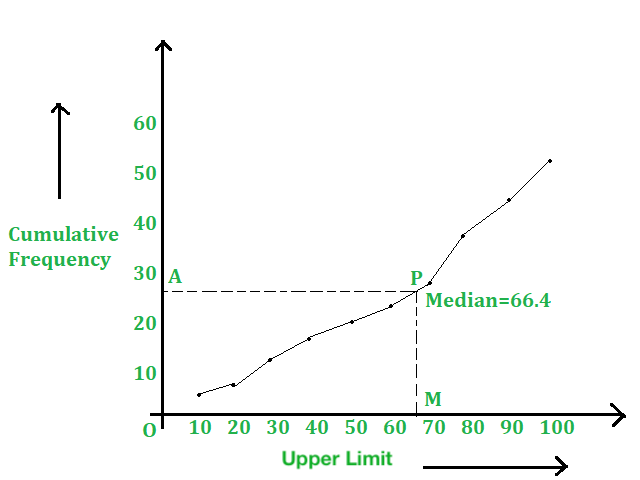(2) More than cumulative frequency curve

As we know that the cumulative frequency curves are created using the cumulative frequencies so, in more than cumulative frequency curve, the frequencies of succeeding class or interval are added to the current class or interval frequency. You can create more than cumulative frequency by subtracting the frequency of the second-class from the first class and so on. For example:

In the above table, we have intervals and frequencies now we are going to find more than the cumulative frequency:

How to draw more than cumulative frequency curve:

In this case, we use the lower limit of the classes to draw the curve. Now, the step-by-step process of plotting a more than Cumulative Frequency curve:

1. Take a graph paper and mark the lower class limits along the x-axis and the corresponding cumulative frequencies along the y-axis.
2. Join these points successively by line segments, we will get a polygon, known as a cumulative frequency polygon.
3. Join these points successively by a smooth curve, we will get a curve, known as cumulative frequency graph.
4. We assume that P be the point of intersection of less than’ and ‘more than curves. Draw PM ⊥ to the y-axis, cutting x-axis at M.
5. Then, median = length of OM.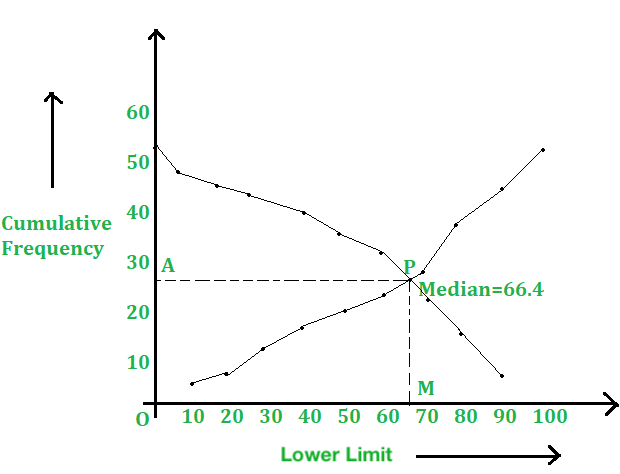### Sample Problem

Question 1. Following is the age distribution of group students. Now, draw the cumulative frequency curve of less than type and find the median value.

Solution:

For the given table, we have to prepare the more than series as shown below:

On a graph paper, take the scale

Along the x-axis: 5 small div. = 1.

Along the y-axis: 1 small div. = 10.

And, plot all the points A(5, 36), B(6, 78), C(7, 130), D(8, 190), E(9, 258), F(10, 342), G(11, 438),

H(12, 520), I(13, 586), J(14, 634), K(15, 684) and L(16, 700).

Join these points successively with a freehand, we will get the cumulative frequency curve or an ogive.

Here, N = 700 ⇒ N/2 = 350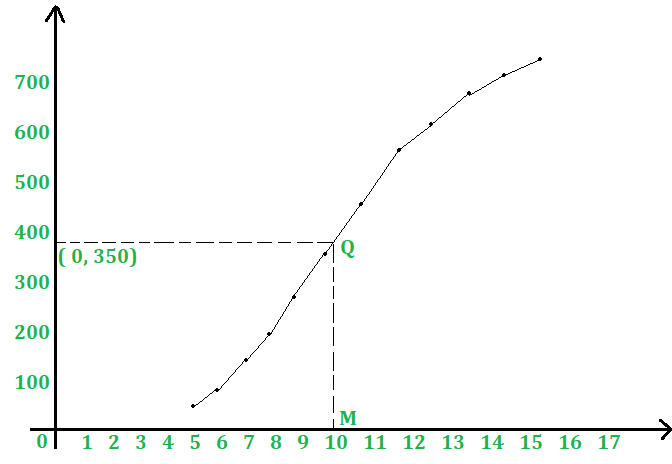Take a point P(0, 350) on the y-axis and draw PQ|| x-axis, meeting the curve at Q. Draw QM 1 x-axis, intersecting x-axis at M. Then, OM = 10 units.

Hence, median = 10.

Question 2. For the given frequency distribution, draw a cumulative frequency graph of more than type and find the median value.

Solution:

For the given table, we have to prepare the more than series as shown below:

Scale: Along the x-axis, 10 small div. = 5.

Along the y-axis, 1 small div.= 1.

Plot all the points A(5, 100), B(10, 95), C(20, 80), D(30, 60), E(40, 37), F(50, 20) and G(60, 9).

Join AB, BC, CD, DE, EF and FG with a freehand, and we will get the required curve, as shown in below figure.

Here, N = 100

⇒ N/2 = 50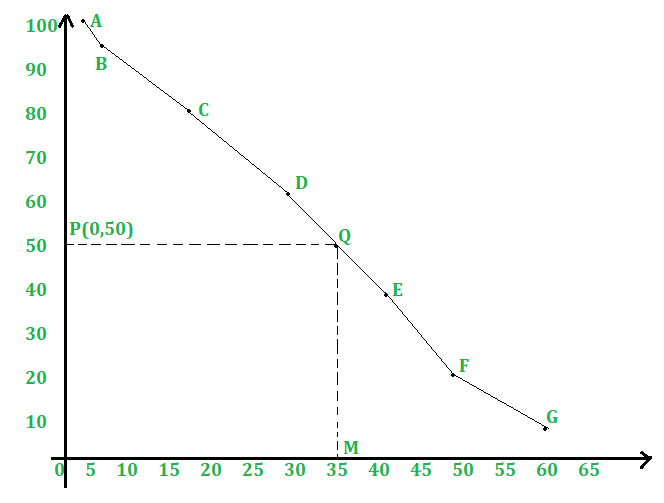From P(0, 50) draw PQ || x-axis, meeting the curve at Q. Draw QM ⊥ OZ, meeting x-axis at M. Clearly, OM = 35 units

Hence, median = 35.

Question 3. The following table gives the production yield of rice of 100 farms of a village:

Draw a cumulative frequency graph of more than type.

Solution:

For the given table, we have to prepare the more than series as shown below:

Scale: Along the x-axis, 1 small div.= 1

Along the y-axis, 1 small div. = 1

On a graph paper, plot all the points A(40, 100), B(45, 96), C(50, 90), D(55, 74), E(60, 54) and F(65, 24).

Join AB, BC, CD, DE and EF with a free hand, and we will get a More Than Ogive.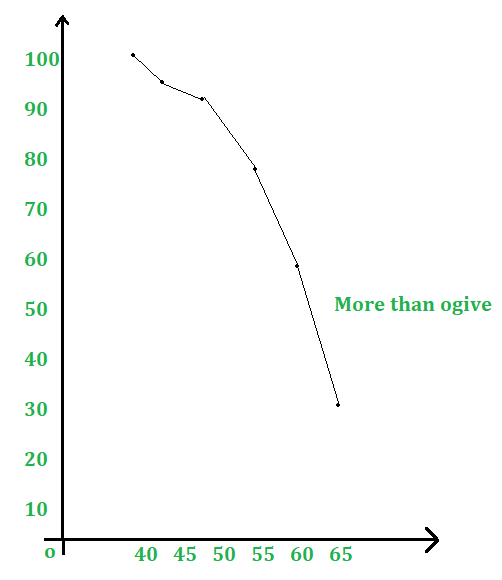Question 4. During the medical checkup of 35 students of a college their weights were recorded as follows:

Draw a less than and a more than type ogive from the given data. Hence, find the median weight from the graph.

Solution:

(i) Less than Series:

For the given table, we have to prepare the less than series as shown below:

Scale: Along the x-axis, 5 small div. = 1 kg.

Along the y-axis, 10 small div.= 5 kg.

Plot all the points A(40, 3), B(42, 5), C(44, 9), D(46, 14), E(48, 28), F(50, 32) and G(52, 35).

Join AB, BC, CD, DE, EF and FG with a free hand to get the curve ‘Less Than Series’.

(ii) More than Series:

For the given table, we have to prepare the more than series as shown below:

Now plot the points on the same graph: P(38,35), Q(40, 32), R(42, 30), S(44, 26), T(46, 21), U(48, 7) and V(50,3)

and join PQ, QR, RS, ST, TU and UV with a free hand to get ‘More Than Series’.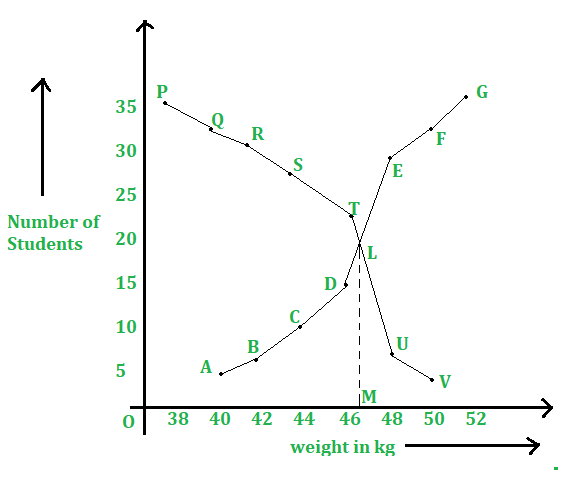The two curves intersect at the point L. Draw LM ⊥ OX.

Hence, median weight = OM = 46.5 kg.

My Personal Notes arrow_drop_up
Related Tutorials